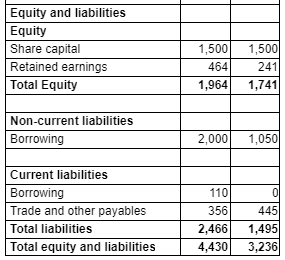# The gearing saga that will never end.

So my students are preparing for their CDMT exam in a couple of weeks time. I have already spoken with them regarding the astonishing decision by the AAT to have different calculations for gearing for each unit of the qualification. I feel like they're slowly getting their heads around the formula used in CDMT (and CTRM).

I am now faced with the fact that the AAT isn't following its own prescribed formula for the mock exams, and therefore I can only assume, the live assessments.

The formula specified by the AAT in their latest qualification specification is as follows:
Gearing ratio can be calculated as either total debt/ (total debt + total equity) x 100% which
is the preferred method, or total debt/total equity x 100%. In CDTM, total debt includes
current and non-current liabilities (short and long-.term borrowings)

In their mock exams, I have attached an image showing the data students face.The AAT have now started using the formula Total borrowings / Total Equity + Total borrowings x 100% to get to their model answers (ignoring other current liabilities). I find it absolutely ridiculous that a) they don't actually use their own formula (ie current liabilities should include all current liabilities surely?) and b) to confuse students further they actually show students what the total liabilities figure is and the total liabilities + equity figure, deliberately misleading in my opinion.

It is embarrassing having to face students and try to justify the plethora of formulas for Gearing that are used in their exams. They have enough to worry about. I am beyond frustrated.

• Hi

I agree whole-heartedly.

The way I've put it to my learners to help them understand why this formula has been used is that they're using interest bearing debt rather than all current liabilities which, when you think about it makes sense - especially when we also use interest cover as thsi would be affected by an increase in interest bearing debt.

I hope (think) this makes sense.

Good luck

Mike
Michael JH Webster AATQB FMAAT
• In Task 7g of CTRM Sample 2 the formula used is the traditional one:

total debt/ (total debt + total equity) x 100% total debt includes
current borrowing (e.g. o/d and current portion of loan) and non-current liabilities

But the specification uses:

total debt/ (total debt + total equity) x 100% which
is the preferred method, or total debt/total equity x 100%. In CDTM, total debt includes
current and non-current liabilities (short and long-.term borrowings)

My student sat CTRM on Thursday and had to calculate gearing and put answer in box. No opportunity to show workings. Fingers crossed that she passed and didn't narrowly fail. Am still waiting for responses from AAT
• Can we please have clarification from the AAT as to the calculation of gearing for each of the relevant units? If it is:

Total debt/(total debt + equity) then please define total debt.